# NCERT Solutions For Class 9 Math Chapter – 7 Exercise – 7.2

NCERT Solutions For Class 9 Math Chapter – 7 Exercise – 7.2

Q1. In an isosceles triangle ABC with AB = AC the bisectors of ∟B and ∟C intersect each other at O Join A to O

Show that:

• OB = OC      (ii) AO bisects ∟A.

Solution:-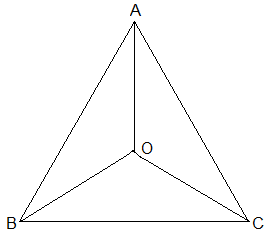In ∆ABC , AB = AC

∟ABC = ∟ACB  [ Angles opposite to equal sides are equal].

= 1/2 ∟ABC = 1/2∟ACB

∟OBC = ∟OCB [ OB and OC are bisectors of ∟ABC and ∟ACB respectively.]

OC = OB [Sides opposite to equal angles of a triangle are equal.]

• Consider triangles AOB and AOC,

OC

= OB [From (I)]

AO = OA [Common]

∆AOB  ∆AOC [SSS rule]

∟OAB = ∟OAC [CPCT]

= AO bisects ∟BAC.

Q2. In ABC , AD is the perpendicular bisector of BC Show that ABC is an isosceles triangle in which AB = AC.

Solution:-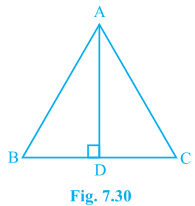BD = BC

AB = AC.

Therefore ∆ABC is an isosceles triangle with AB = AC. [CPCT]

Q3. ABC is an isosceles  triangle in which altitudes BE and CF are drawn to equal sides AC and AB respectively Show that these altitudes are equal.

Solution:-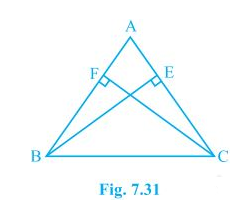In ∆BFC and BCE.

FBC = ∟ECB.

BC = CB

BFC = ∟CEB

BCF  CBE.

CF = BE.

Q4. ABC is a triangle in which altitudes BE and CF to sides AC and AB are equal Show that:

• ABE ACF
• AB = AC ABC is an isosceles triangle.

Solution:-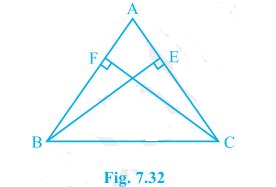• In triangle ABE and ACF,

BE = CF

∟A = ∟A

∟AEB = ∟AFC

∆ABE  ∆ACF [AAS Rule]

• Since ∆ABE ∆ACF

Hence AB = AC

∆ABC is a isosceles.

Q5. ABC and DBC are two isosceles triangles on same base BC Show that ABD = ACD

Solution:-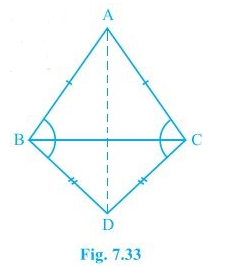In triangle ABD and ACD,

AB = AC

BD = CD

∆ABD  ∆ACD

∟ABD = ∟ACD

Q6. ∆ABC is an isosceles triangle in which AB = AC Side BA is produced to D Such that AD = AB Show that ∟BCD is a right angle.

Solution:-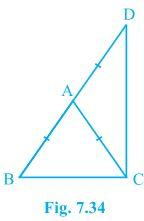AB = AC  [Given]

1 = ∟2  (I)

[Angles opposite to equal sides of a triangle are equal.]

And

∟3 = ∟4

[Angles opposite to equal sides of a triangle are equal.]

In ∆ DBC,

DBC + ∟BCD + ∟CDB = 1800

[ Sum of angles of a triangle is 1800]

= ∟1 + ∟2 + ∟3 + ∟4 = 1800

= ∟2 + ∟2 + ∟3 + ∟3 = 1800

2(∟2 + ∟3) = 1800

2 + ∟3 = 900

Q7. ABC is a right angled triangle in which ∟A = 900 and AB = AC Find ∟B and ∟C.

Solution:-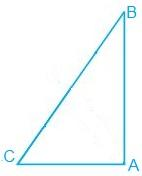As AB = AC

B = ∟C     [ Angels opposite to equal sides of a triangle are equal].

In ∆ABC ,

∟A + ∟B + ∟C = 1800

2∟B = 900

∟B = 450

∟B = ∟C = 450

Q8. Show that the angels of an equilateral triangle are 600 each.

Solution:-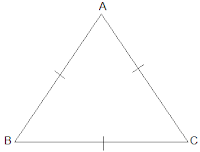In ∆ABC is equilateral

AB = BC = CA

AB = BC

∟A = ∟C

[ Angles opposite to equal sides of a triangle are equal].

BC = AC = ∟A = ∟B

∟A =  ∟B = ∟C

In ∆ABC  ,

∟A + ∟B + ∟C = 1800

[ Sum of angels of a triangle is 1800.]

= ∟A + ∟A + ∟A = 1800

3∟A = 1800

∟A = 600

∟A = ∟B = ∟C = 600